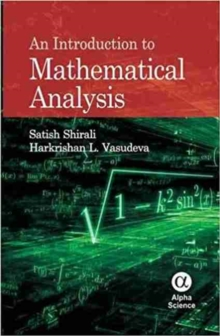Supporting your high street Find out how »
• My Account# An Introduction to Mathematical Analysis Hardback

#### Description

AN INTRODUCTION TO MATHEMATICAL ANALYSIS is an elementary text on the theory of functions of one real variable and is intended for students with a good understanding of calculus.

It is supposed to replace traditional and outmoded courses in mathematical analysis. The book begins with material on the real number system as a Dedekind complete ordered field, continuous functions, sequences and series of constant terms as well as of functions.

Pointwise and uniform convergence of series of functions, power series, treatment of trigonometric and exponential functions in terms of series are discussed.

A detailed treatment of differentiation, including differentiation of uniform limits of sequences is provided.

Also included is an example of a continuous nowhere differentiable function.

There is an exhaustive treatment of both Riemann integration and Riemann-Stieltjes integration.

Some features of this work, such as why Dedekind completeness is necessary for ensuring that nonconstant functions cannot have derivative zero everywhere on an interval, irrationality of evaluation of without using multiple integration are normally not found in textbooks at this level. There are several illustrative and worked examples, and a large number of problems with solutions (available on a compact disc).

#### Information

• Format: Hardback
• Pages: 386 pages
• Publisher: Alpha Science International Ltd
• Publication Date:
• Category: Calculus & mathematical analysis
• ISBN: 9781842658475

£39.95

£33.95£29.99

£25.49

£55.00

£46.75

£44.99

£38.24

£40.00

£34.00